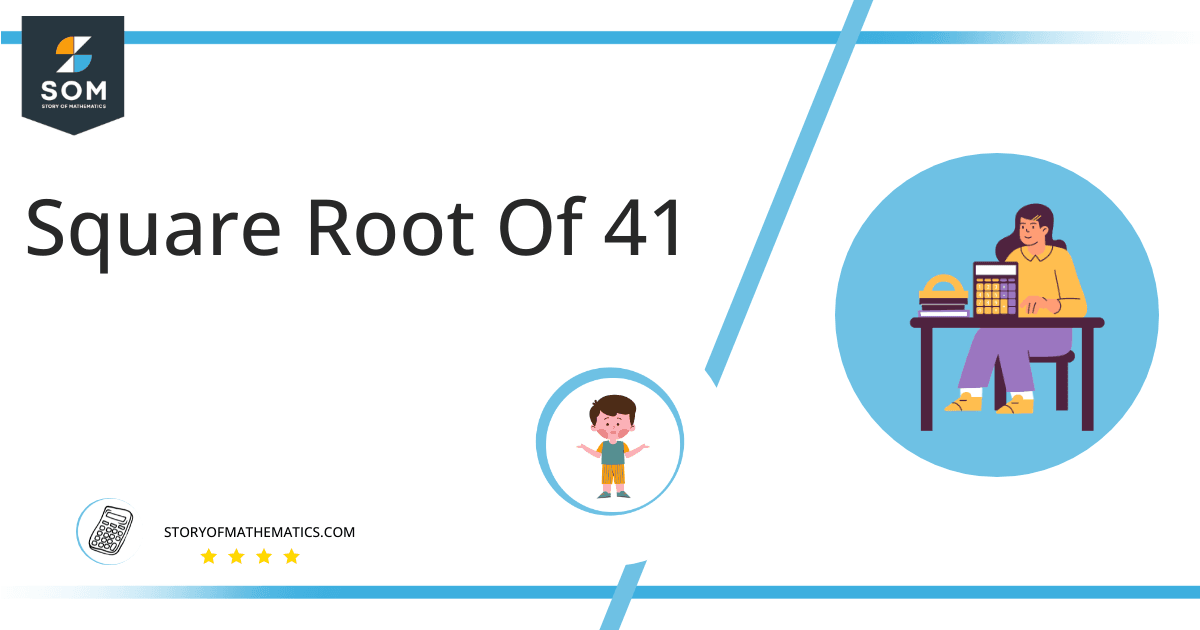# Square Root of 41 + Solution With Free StepsTo find the square root of the number 41, we have to write it under the symbol , which gives √41 which is equal to 6.40. The square root of a number is always less than the number itself for example in this scenario 6.40 which is the square root of 41 is less than the number 41.

In this article, we will analyze and find the square root of 41 using various mathematical techniques, such as the approximation method and the long division method.

## What Is the Square Root Of 41?

The square root of the number 41 is 6.40.

The square root can be defined as the quantity that can be doubled to produce the square of that similar quantity. In simple words, it can be explained as:

√41 = √(6.40 x 6.40)

√41 = √(6.40)$^2$

√41 = ±6.40

The square can be canceled with the square root as it is equivalent to 1/2; therefore, obtaining 6.40. Hence 6.40 is 41’s square root. The square root generates both positive and negative integers.

## How To Calculate the Square Root of 41?

You can calculate the square root of 41 using any of two vastly used techniques in mathematics; one is the Approximation technique, and the other is the Long Division method.

The symbol √ is interpreted as 41 raised to the power 1/2. So any number, when multiplied by itself, produces its square, and when the square root of any squared number is taken, it produces the actual number.

Let us discuss each of them to understand the concepts better.

### Square Root by Long Division Method

The process of long division is one of the most common methods used to find the square roots of a given number. It is easy to comprehend and provides more reliable and accurate answers. The long division method reduces a multi-digit number to its equal parts.

Learning how to find the square root of a number is easy with the long division method. All you need are five primary operations- divide, multiply, subtract, bring down or raise, then repeat.

Following are the simple steps that must be followed to find the square root of 41 using the long division method:

### Step 1

First, write the given number 41 in the division symbol, as shown in figure 1.

### Step 2

Starting from the right side of the number, divide the number 41 into pairs such as 41.

### Step 3

Now divide the digit 41 by a number, giving a number either 41 or less than 41. Therefore, in this case, the remainder is 5 , whereas the quotient is 6.

### Step 4

After this, bring down the next pair 00. Now the dividend is 500. To find the next divisor, we need to double our quotient obtained before. Doubling 6 gives 12; hence consider it as the next divisor.

### Step 5

Now pair 6 with another number to make a new divisor that results in $\leq$ 500 when multiplied with the divisor. If the number is not a perfect square, add pair of zeros to the right of the number before starting division.

### Step 6

Adding 4 to the divisor and multiplying 124 with 4 results in 496 $\leq$ 500. The remainder obtained is 4. Move the next pair of zeros down and repeat the same process mentioned above.

### Step 7

Keep on repeating the same steps till the zero remainder is obtained or if the division process continues infinitely, solve to two decimal places.

### Step 8

The resulting quotient of 6.40 is the square root of 41. Figure 1 given below shows the long division process in detail:### Square Root by Approximation Method

The approximation method involves guessing the square root of the non-perfect square number by dividing it by the perfect square lesser or greater than that number and taking the average.

The given detailed steps must be followed to find the square root of 41 using the approximation technique.

### Step 1

Consider a perfect square number 36 less than 41.

### Step 2

Now divide 41 by √36.

41 ÷ 6 = 6.83

### Step 3

Now take the average of 6 and 6.83. The resulting number is approximately equivalent to the square root of 41.

(6+6.83) ÷ 2 = 6.41### Important points

• The number 41 is not a perfect square.
• The number 41 is a rational number.
• The number 41 can be split into its prime factorization.

## Is Square Root of 41 a Perfect Square?

The number 41 is not a perfect square. A number is a perfect square if it splits into two equal parts or identical whole numbers. If a number is a perfect square, it is also rational.

A number expressed in p/q form is called a rational number. All the natural numbers are rational. A square root of a perfect square is a whole number; therefore, a perfect square is a rational number.

A number that is not a perfect square is irrational as it is a decimal number. As far as 41 is concerned, it is not a perfect square. It can be proved as below:

Factorization of 41 results in 41 x 1.

Taking the square root of the above expression gives:

= √(41 x 1)

= (41x 1)$^{1/2}$

= 6.40

This shows that 41 is not a perfect square as it has decimal places; hence it is an irrational number.

Therefore the above discussion proves that the square root of 41 is equivalent to 6.40.Images/mathematical drawings are created with GeoGebra.